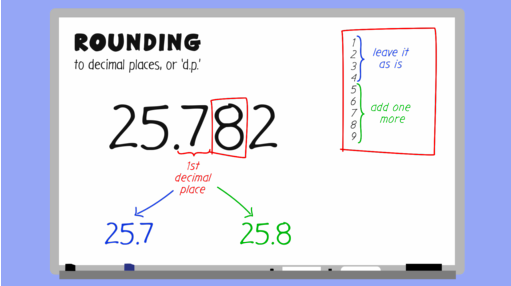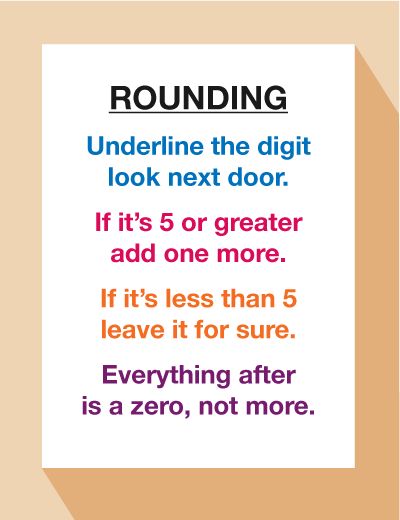Science, Maths & Technology

### Become an OU studentEveryday maths 2

Start this free course now. Just create an account and sign in. Enrol and complete the course for a free statement of participation or digital badge if available.

# 2.3 Rounding to a degree of accuracy

Watch the short video below to see an example of how to round to one, two and three decimal places.Interactive feature not available in single page view (see it in standard view).Figure _unit2.2.2 Figure 3 A rounding rhyme

## Activity _unit2.2.3 Activity 5: Rounding skills

Practise your rounding skills by completing the below.

1. What is 24.638 rounded to one decimal place?

2. What is 13.4752 rounded to two decimal places?

3. What is 203.5832 rounded to two decimal places?

4. What is 345.6795 rounded to three decimal places?

1. 24.6

2. 13.48

3. 203.58

4. 345.680

## Summary

In this section you have learned:

• how to decide whether an answer to a division calculation needs to be to rounded up or down, depending on the context of the question

• how and when to use rounding to approximate an answer to a calculation

• how to round an answer to a given degree of accuracy – e.g. rounding to two decimal places.